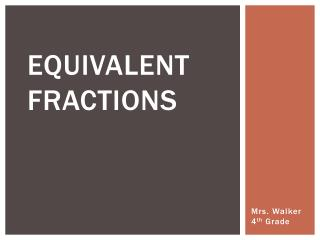DownloadDownload PresentationEquivalent Fractions

# Equivalent Fractions

Télécharger la présentation## Equivalent Fractions

- - - - - - - - - - - - - - - - - - - - - - - - - - - E N D - - - - - - - - - - - - - - - - - - - - - - - - - - -
##### Presentation Transcript

1. Equivalent Fractions Mrs. Walker 4th Grade

2. Vocabulary: Equivalent Fractions Equivalent fractions are fractions that name the same amount. 2 4 = 8 4

3. Creating Equivalent Fractions Multiply the numerator and denominator by the samenumber. We can choose any number to multiply by. Let’s multiply by 2. 3 x 2 6 = So, 3/5 is equivalent to 6/10. x 2 10 5

4. Creating Equivalent Fractions Divide the numerator and denominator by a commonfactor. (It has to be a common factor to work with division.) To divide we MUST use a common factor. What number is a common factor of 6 ? 10 6 ÷ 2 3 So, 3/5 is equivalent to 6/10. = ÷ 2 5 10

5. For larger numbers, make equivalent fractions using division. Remember, divide by a common factor. 28 ÷7 4 = 35 ÷7 5 Think to yourself… What number can I divide into both 28 and 35? In this example, we can divide both numbers by 7. 28/35 is equivalent to 4/5.

6. Vocabulary: Simplest Form Fractions are in simplestform when the numerator and denominator do not have any common factors besides 1. Examples of fractions that are in simplest form: 4 2 3 5 11 8

7. Vocabulary: Simplify Simplify means to reduce a fraction to it’s simplest form.

8. Writing Fractions in Simplest Form. • Find the greatestcommonfactor (GCF) of the numerator and denominator. • Divide both numbers by the GCF.

9. Example: 20 5 ÷ 4 = Simplest Form 28 ÷ 4 7 20 28 We will divide by 4. 20: 1, 2, 4, 5, 10, 20 28: 1, 2, 4, 7, 14, 28 1 x 20 2 x 10 4 x 5 1 x 28 2 x 14 4 x 7 Common Factors: 1, 2, 4 GCF: 4

10. Homework Time